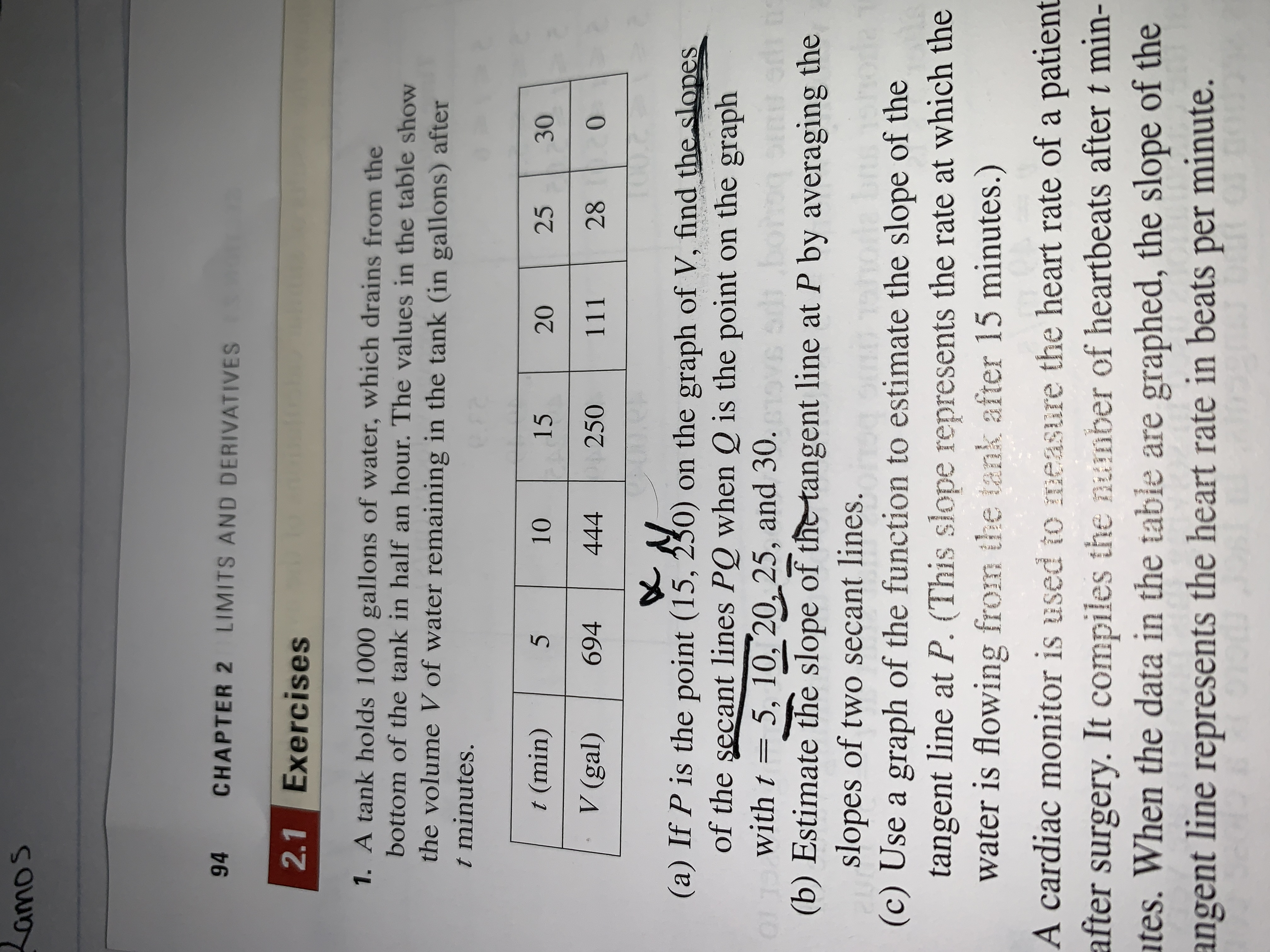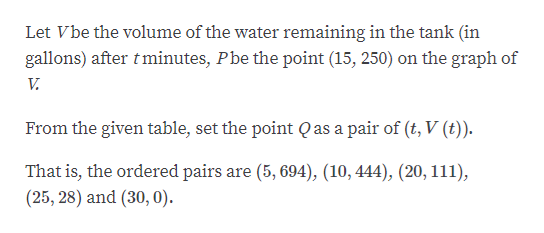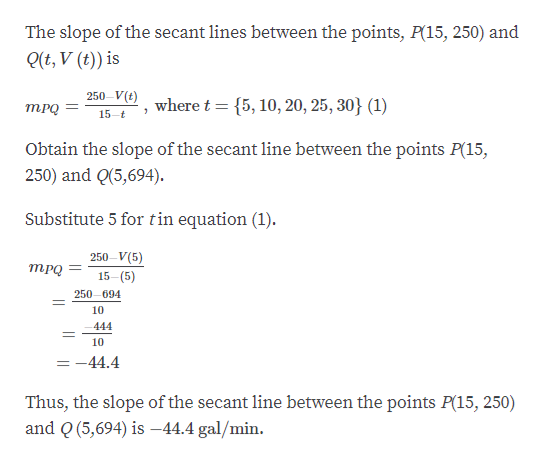# amos94CHAPTER 2 LIMITS AND DERIVATIVES2.1 Exercises1. A tank holds 1000 gallons of water, which drains from thebottom of the tank in half an hour. The values in the table showthe volume V of water remaining in the tank (in gallons) aftert minutes.t (min)51015252030V (gal)69428 0444250111(a) If P is the point (15, 230) on the graph of V, find the slopesof the secant lines PQ when Q is the point on the graphwith t 5, 10, 20 25, and 30.sdi b01(b) Estimate the slope of the tangent line at P by averaging theslopes of two secant lines.(c) Use a graph of the function to estimate the slope of thetangent line at P. (This slope represents the rate at which thewater is flowing from the tank after 15 minutes.)0A cardiac monitor is used to measure the heart rate of a patientafter surgery. It compiles the number of heartbeats after t min-tes. When the data in the table are graphed, the slope of theangent line represents the heart rate in beats per minute.01t1909

Question
7 views

I don't know understand one and it's parts.help_outlineImage Transcriptioncloseamos 94 CHAPTER 2 LIMITS AND DERIVATIVES 2.1 Exercises 1. A tank holds 1000 gallons of water, which drains from the bottom of the tank in half an hour. The values in the table show the volume V of water remaining in the tank (in gallons) after t minutes. t (min) 5 10 15 25 20 30 V (gal) 694 28 0 444 250 111 (a) If P is the point (15, 230) on the graph of V, find the slopes of the secant lines PQ when Q is the point on the graph with t 5, 10, 20 25, and 30. sdi b 01 (b) Estimate the slope of the tangent line at P by averaging the slopes of two secant lines. (c) Use a graph of the function to estimate the slope of the tangent line at P. (This slope represents the rate at which the water is flowing from the tank after 15 minutes.) 0 A cardiac monitor is used to measure the heart rate of a patient after surgery. It compiles the number of heartbeats after t min- tes. When the data in the table are graphed, the slope of the angent line represents the heart rate in beats per minute. 01 t 1909 fullscreen
check_circle

Step 1

Given,help_outlineImage TranscriptioncloseLet Vbe the volume of the water remaining in the tank (in gallons) after tminutes, Pbe the point (15, 250) on the graph of V. From the given table, set the point Qas a pair of (t, V (t)) That is, the ordered pairs are (5,694), (10, 444), (20, 111), (25, 28) and (30, 0) fullscreen
Step 2

Find the slope of the secant lines PQ when Q is the point on the graph with t = 5, 10, 20, 25 and 30.help_outlineImage TranscriptioncloseThe slope of the secant lines between the points, P(15, 250) and (t, V (t)) is 250-V) where t={5,10,20, 25,30} (1) тРо 7 15 t Obtain the slope of the secant line between the points P(15, 250) and Q(5,694) Substitute 5 for tin equation (1). 250-V(5) тPо 15-(5) 250-694 10 -444 10 - -44.4 Thus, the slope of the secant line between the points P(15, 250) and Q (5,694) is -44.4 gal/min fullscreen
Step 3

Now, obtain the other slopes by substituting Q (10, ...

### Want to see the full answer?

See Solution

#### Want to see this answer and more?

Solutions are written by subject experts who are available 24/7. Questions are typically answered within 1 hour.*

See Solution
*Response times may vary by subject and question.
Tagged in

### Derivative